In :
import matplotlib.pylab as plt
import numpy as np
%matplotlib inline
from IPython.display import Image


Part of the cells are copyed from Fernando Perez talk

## The Lifecycle of a Scientific Idea (schematically)¶

1. **Individual** exploratory work
2. **Collaborative** development
3. **Parallel** production runs (HPC, cloud, ...)
4. **Publication & communication** (with reproducible results!)
5. **Education**
6. Goto 1.

# IPython notebook¶

Since the 0.12 release, IPython provides a new rich text web interface - IPython notebook. Here you can combine:

### Code execution¶

In :
print('I love Python 2000!')

I love Python 2000!


### Text (Markdown)¶

IPython website.

List:

Code:

print('hello world')

### $\LaTeX$ equations¶

$$\int_0^\infty e^{-x^2} dx=\frac{\sqrt{\pi}}{2}$$$$F(x,y)=0 ~~\mbox{and}~~ \left| \begin{array}{ccc} F''_{xx} & F''_{xy} & F'_x \\ F''_{yx} & F''_{yy} & F'_y \\ F'_x & F'_y & 0 \end{array}\right| = 0$$

### Plots¶

In :
x = [1,2,3,4,5,6,7]
plt.plot(x)

Out:
[<matplotlib.lines.Line2D at 0x7f38e85aad50>]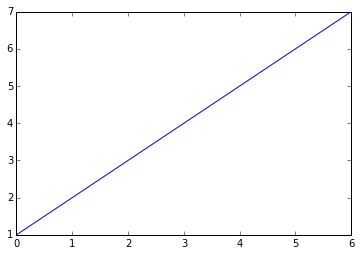### Interactive plots¶

In :
from IPython.html.widgets import *

In :
x=np.linspace(0,1,100)
def pltsin(f, a):
plt.plot(x,a*np.sin(2*np.pi*x*f))
plt.ylim(-10,10)

In :
interact(pltsin, f=(1,10,0.1), a=(1,10,1));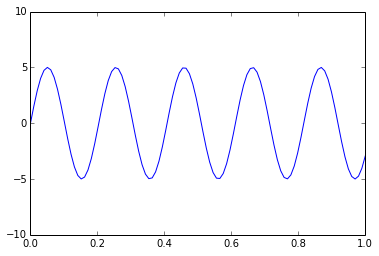### Images¶## It's not python specific system¶

### Accessing the underlying operating system¶

You can access system functions by typing exclamation mark.

In :
!pwd

/home/magik/CSC/eddy_ipython


If you already have some netCDF file in the directory and ncdump is installed, you can for example look at its header.

In [ ]:
#download some data
!wget ftp://ftp.cdc.noaa.gov/Datasets/ncep.reanalysis.dailyavgs/surface/air.sig995.1981.nc

In :
!ncdump -h air.sig995.1981.nc

netcdf air.sig995.1981 {
dimensions:
lon = 144 ;
lat = 73 ;
time = UNLIMITED ; // (365 currently)
variables:
float lat(lat) ;
lat:units = "degrees_north" ;
lat:actual_range = 90.f, -90.f ;
lat:long_name = "Latitude" ;
lat:standard_name = "latitude" ;
lat:axis = "Y" ;
float lon(lon) ;
lon:units = "degrees_east" ;
lon:long_name = "Longitude" ;
lon:actual_range = 0.f, 357.5f ;
lon:standard_name = "longitude" ;
lon:axis = "X" ;
double time(time) ;
time:long_name = "Time" ;
time:delta_t = "0000-00-01 00:00:00" ;
time:avg_period = "0000-00-01 00:00:00" ;
time:standard_name = "time" ;
time:axis = "T" ;
time:units = "hours since 1800-01-01 00:00:0.0" ;
time:actual_range = 1586616., 1595352. ;
float air(time, lat, lon) ;
air:long_name = "mean Daily Air temperature at sigma level 995" ;
air:units = "degK" ;
air:precision = 2s ;
air:least_significant_digit = 1s ;
air:GRIB_id = 11s ;
air:GRIB_name = "TMP" ;
air:var_desc = "Air temperature" ;
air:dataset = "NCEP Reanalysis Daily Averages" ;
air:level_desc = "Surface" ;
air:statistic = "Mean" ;
air:parent_stat = "Individual Obs" ;
air:missing_value = -9.96921e+36f ;
air:actual_range = 191.06f, 317.13f ;
air:valid_range = 185.16f, 331.16f ;

// global attributes:
:Conventions = "COARDS" ;
:title = "mean daily NMC reanalysis (1981)" ;
:description = "Data is from NMC initialized reanalysis\n",
"(4x/day).  These are the 0.9950 sigma level values." ;
:platform = "Model" ;
:references = "http://www.esrl.noaa.gov/psd/data/gridded/data.ncep.reanalysis.html" ;
:history = "created 95/02/06 by Hoop (netCDF2.3)\n",
"Converted to chunked, deflated non-packed NetCDF4 2014/09" ;
}


### Example of cdo use:¶

Get some information about file with cdo.

In :
!cdo nyear air.sig995.1981.nc

1
cdo nyear: Processed 1 variable over 365 timesteps ( 0.01s )


Create monthly mean

In :
!cdo monmean air.sig995.1981.nc air.sig995.1981_mm.nc

cdo monmean: Processed 3836880 values from 1 variable over 365 timesteps ( 0.24s )


Let's plot it first with good old GrADS:

In :
%%writefile sc.gs
'sdfopen air.sig995.1981_mm.nc'
'd air'
'printim out.png'
* 'printim out.png white x400 y300'

Overwriting sc.gs

In :
!grads -blxc "run sc.gs" > log
Image('out.png')

Out: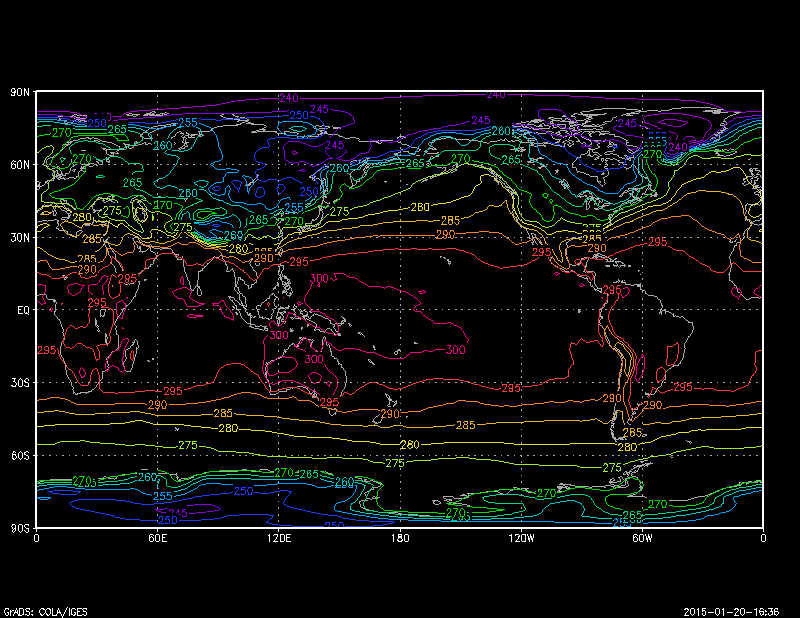### NCL¶

Look a bit old fasioned. Let's try something more advanced, like NCL:

In :
%%writefile nc2.ncl
load "$NCARG_ROOT/lib/ncarg/nclscripts/csm/gsn_code.ncl" load "$NCARG_ROOT/lib/ncarg/nclscripts/csm/gsn_csm.ncl"
begin
air = a->air(1,:,:)                                ; read July zonal winds

wks_type = "png"
wks_type@wkWidth = 800
wks_type@wkHeight = 600

wks = gsn_open_wks(wks_type,"ce")                  ; open a ps file
gsn_define_colormap(wks,"BlAqGrYeOrRe")        ; choose colormap

res                       = True     ; plot mods desired
res@cnFillOn              = True     ; turn on color fill
res@cnLevelSpacingF       = 5      ; contour spacing
res@gsnMaximize = True
plot = gsn_csm_contour_map_ce(wks,air,res)     ; create a default plot
end

Overwriting nc2.ncl

In :
!ncl nc2.ncl > log
!convert -trim +repage ce.png ce1.png
Image('ce1.png')

Out: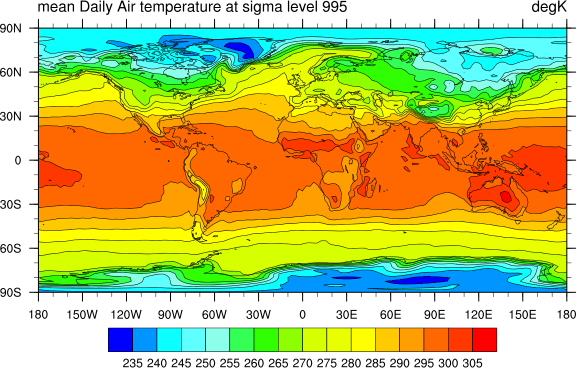### Python and R friends forever¶

Now let's try to do some pointwise analysis of the temperature. First we open our netCDF file in Python

In :
from netCDF4 import Dataset, num2date


Get air variable from netCDF file

In :
f = Dataset('air.sig995.1981.nc')
air = f.variables['air'][:]-273.15


Select two points

In :
air_point = air[:,30,30]
air_point2 = air[:,50,50]

x = range(air_point.shape)

In :
plt.plot(x, air_point, x, air_point2);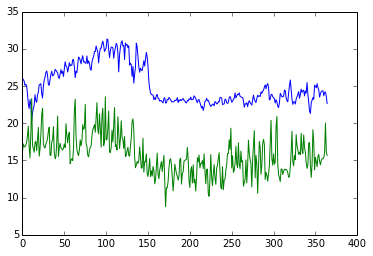Never liked default matplotlib style, and I need to put some shading between the lines. I gues R is a good choice for this.

In :
%load_ext rpy2.ipython

The rpy2.ipython extension is already loaded. To reload it, use:


### Push data to R and plot them¶

In :
%%R -i air_point,air_point2,x

y1 <- air_point
y2 <- air_point2

plot(x,y1,type="l",bty="L",xlab="days",ylab="temperature", ylim=c(5, 35))
points(x,y2,type="l",col="red")
polygon(c(x,rev(x)),c(y2,rev(y1)),col="skyblue")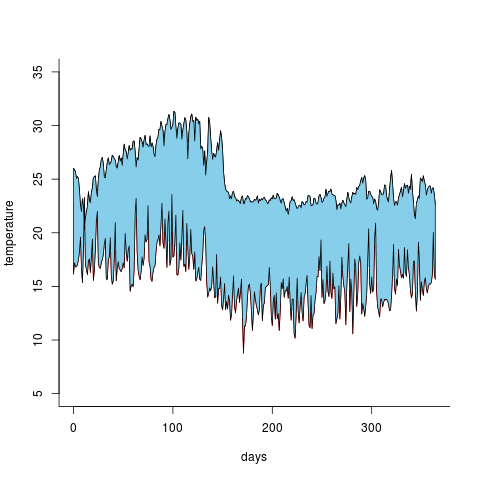Pretty nice :)

### Python and Fortran¶

Let's pretend I forgot how to use numpy arrays and decide to calculate sum of all vlues in air using loop:

In :
air.shape

Out:
(365, 73, 144)
In :
def asum(air):
z = 0
for j in range(air.shape):
for k in range(air.shape):
for l in range(air.shape):
z = z+air[j,k,l]
return z

In :
%time asum(air)

CPU times: user 1.98 s, sys: 8 ms, total: 1.98 s
Wall time: 1.96 s

Out:
17530874.218353271

I want it to be faster. Maybe Fortran will help?

In [ ]:
#%install_ext https://raw.github.com/mgaitan/fortran_magic/master/fortranmagic.py

In :
%load_ext fortranmagic

In :
%%fortran
subroutine sum_fort(a,i,m,n,z)
integer :: j,k,l
integer :: i, m, n
real*8 :: a(i,m,n)
real*8, intent(out) :: z

z=0
do j = 1,i
do k = 1,m
do l= 1,n
z=z+a(j,k,l)
end do
end do
end do

end subroutine sum_fort

In :
%time sum_fort(air)

CPU times: user 64 ms, sys: 8 ms, total: 72 ms
Wall time: 73.8 ms

Out:
17530874.21835327

Lightning fast! And also nice documentation generated automatically:

In :
print sum_fort.__doc__

z = sum_fort(a,[i,m,n])

Wrapper for sum_fort.

Parameters
----------
a : input rank-3 array('d') with bounds (i,m,n)

Other Parameters
----------------
i : input int, optional
Default: shape(a,0)
m : input int, optional
Default: shape(a,1)
n : input int, optional
Default: shape(a,2)

Returns
-------
z : float



## Python is not the only possible core language for IPython notebook¶

Kernels, they are everywhere!
1. IPython "Official".
2. IJulia
4. IFSharp
5. IRuby
6. IGo
7. IScala
8. IMathics
9. IAldor
10. IRKernel
11. IErlang
12. More... (~20 and counting)
In :
%%bash

echo "My shell is:" $SHELL echo "My memory status is:" free  My shell is: /bin/bash My memory status is: total used free shared buffers cached Mem: 3925620 3487912 437708 0 317128 1640752 -/+ buffers/cache: 1530032 2395588 Swap: 3906556 10848 3895708  In : %%perl$variable = 1;
print "The variable has the value of \$variable\n";

The variable has the value of 1


You can write content of the cell to a file with %%writefile (or %%file for ipython < 1.0):